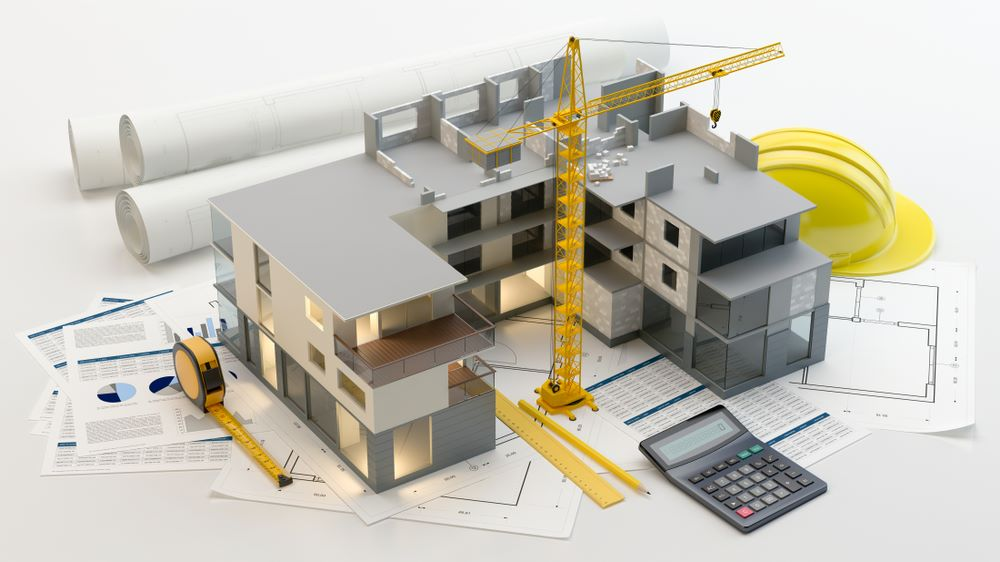# Concrete Calculator

Concrete is a high compressive building material that is strong and durable. It is used in buildings of all kinds. Therefore, it’s essential for builders to correctly estimate the amount of ready mix concrete needed for building a particular structure. Pre-mixed concrete comes in different weights and has different densities.

A concrete calculator helps builders get an idea of the weight and volume of concrete required for a particular area.

For walls, slabs or square footings

• The length, width, height/thickness in feet/inches and quantity needs to be provided
• For columns, holes and round footings
• The height and diameter need to be furnished in feet along with the quantity.
• For circular tubes
• The inner and outer diameter, the length in feet and quantity has to be entered

The total area for which concrete is used is then furnished in cubic yards/cubic feet. It will also let you know how many 40, 60, or 80 pound bags of concrete you will require. As the calculator only gives an estimate of the amount of concrete needed, it’s always a good idea to buy a little more just in case you fall short.

Measure Manage Provides Concrete Calculator Service in Perth Western Australia. Call Us Now at 0489 202 445 or Feel Free to Contact Us

### What our concrete cost estimation calculator does not include:

• Preparation work
• Tools
• Labour
• Transport of materials
• Reinforcement, mesh or joints
• Concrete pumping

### Some tools required for the concrete project:

• Wheel barrows
• Shovels
• Buckets
• Cement mixers
• Levelling tools
• Trowels

Watch this video below where I shown you exactly how to use the paint calculator and explain what the calculation results mean.

To give you the best chance to calculate the paint costs and quantity required for your project.

## FAQ’s

### How to calculate how much concrete I need?

Calculating the amount of concrete, you require can be tricky. Generally, multiply the length x width x depth will give you a quantity but you must consider how much waste there will be and if you will require more than the theoretical amount of concrete.

### How to calculate the cost of how much concrete I need?

Concrete estimating is difficult as a lot of factors must be considered. If you are mixing the concrete yourself then this will take a lot of time compared to bringing in ready mix concrete and pumping it. Each scenario needs to closely analyzed to see what it entails to provide accurate costings.

### How to calculate how many concrete bags I need?

Multiply the length x width x depth to get a meter cubec (m3) quantity. Then divide it by the size of the concrete dry premix bag which are generally around 0.01m3. This will give you the amount of bags you required.